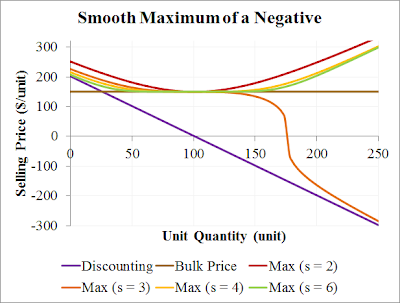## Saturday 15 December 2012

### Smooth Minimum and Maximum

The functions we examine in this post are closely related to p-norms. Lets say we have two equations that describe aspects of a relationship. For example, we might describe the selling price of an item in terms of a discounted price, $$d$$, for small purchases, and a lower bound price, $$u$$, for bulk purchases. In that case, we might have two equations to describe price: $$d(x)=202-2x,$$ and $$u(x)=150,$$ where $$x$$ is the number of units purchased in a single transaction. In this case, it might be fine to simply decide the selling price, $$p$$ as $$p(x) = \max(d(x), u(x)),$$ but what if we want a formula for the selling price that has continuous derivatives? (i.e. is smooth) Well, the formula I found in an attempt to do this is: $$m(x, s) = (d(x)^s + u(x)^s)^{1/s},$$ where $$s$$ is the 'strength' of the maximisation.

The above formula is basically a p-norm (and is the Euclidean norm when $$s$$ = 2). Here's a chart of it in action on $$d$$ and $$u$$ with $$s$$ set to 2, 4, 6 and 8:Example of a smooth maximum function for combining formulas.
The chart shows that a higher strength, $$s$$ leads to a 'tighter' maximum. In the limit as $$s$$ goes to infinity, we actually obtain the plain $$\max$$ function. But what happens at higher unit quantities? Specifically, what happens when one of our inputs is negative? Well, that's shown here:Example of the effect of a negative input on the smooth maximum.

That's not so good. The even strengths stay above both inputs, but diverge further and further above the intended maximum. The odd strengths (only one shown) start to trend towards the negative input, because towards the right, it is the largest in absolute value.

Before we attempt to deal with this issue, let's look at another interesting behaviour. Let's consider negative strengths. This chart shows strengths of -2, -3, -4 and -5:A negative strength produces a smooth minimum function!

That's a convenient way to take the continuous minimum! And what happens when we feed in negative inputs? Well, it gets a little crazy:A smooth minimum of a negative input does not look pretty.

So then, how might we deal with negative inputs? One way would be to transform inputs from the full number line onto just the positive half. To do that we turn to exponentiation. So here's the updated continuous maximum/minimum function, generalised to any number of functions: $$m(x, s) = \ln_b\left(\left[ \sum_{i=1}^q (b^{f_i(x)})^s \right]^{1/s}\right),$$ where $$b$$ is the base of exponentiation. So, let's test it. I initially tried $$w = e$$, but ran into overflow issues, and instead have used the value 1.01. Here is the maximum and minimum of positive and negative inputs, with $$s$$ being 2, 5, -2 and -5:A smooth minimum and maximum function that works with negative inputs.

One final thing. To use this in Lisp on individual values, evaluate this (with MathP):

(defun smooth-min-max (b s &rest vals)
"Smooth minimum (s < -1) or maximum"
#M log(reduce{#'+ vals :key fn(v) (b^v)^s}^(1/s) b))

And to construct a function that minimises or maximises other functions, try something like this:

(defun smooth-min-max-lambda (b s &rest funcs)
"Returns a function that calculates a smooth min/max of the

given base and strength. If b > 1, positive s does maximisation,
negative s minimisation. If 0 < b < 1, it is reversed."
(assert (or (< 0 b 1) (> b 1)))
(assert (not (zerop s)))
#M fn(&rest args) log(reduce(#'+ funcs :key fn(f) (b^apply(f args))^s)^(1/s) b))

Enjoy!

1.This comment has been removed by the author.

2.Your Function is flawed consider b^0 = 1 therefore say you have f1(x) = 0, f2(x) = 0 and f3(x) = 0 your function reduced to (1^s+1^s+1^s)^(1/s) = 3^(1/s) != 1 therefor log_b(3^(1/s)) != 0, note this error is only significant near 0, i.e. as any single value -> inf the error -> 0,
if you set s=1, then it can be corrected by subtracting q-1 from the sum

3.In case x values where 0 the gradient will be divided by 0. how this could be solved without adding constant value.

4.188bet - THTOP Bet
188bet: 188bet.me; 온카지노 188bet.me; 188bet 188bet.me. 188bet.me; 188bet.me. 188bet.me; 188bet.me. 188bet.me.